Courses

# Quantum Mechanics In Three Dimensions – MSQ

## 10 Questions MCQ Test Topic wise Tests for IIT JAM Physics | Quantum Mechanics In Three Dimensions – MSQ

Description
This mock test of Quantum Mechanics In Three Dimensions – MSQ for Physics helps you for every Physics entrance exam. This contains 10 Multiple Choice Questions for Physics Quantum Mechanics In Three Dimensions – MSQ (mcq) to study with solutions a complete question bank. The solved questions answers in this Quantum Mechanics In Three Dimensions – MSQ quiz give you a good mix of easy questions and tough questions. Physics students definitely take this Quantum Mechanics In Three Dimensions – MSQ exercise for a better result in the exam. You can find other Quantum Mechanics In Three Dimensions – MSQ extra questions, long questions & short questions for Physics on EduRev as well by searching above.
*Multiple options can be correct
QUESTION: 1

### Which of the following correspond to the possible states of a hydrogen atom with n = 2. (States are designated as ψnlm.)

Solution: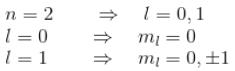The correct answers are: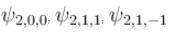*Multiple options can be correct
QUESTION: 2

### For particle in a 3d  box of length a. The state with energy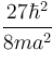has :

Solution: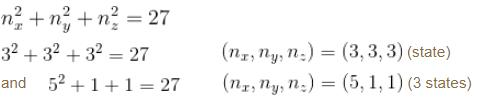The correct answers are: (nxnynz) taking values 1, 1, 5, (nxnynz) taking values 3, 3, 3, is 4 fold degenerate

*Multiple options can be correct
QUESTION: 3

### For a particle in a 3 dimensional box

Solution:

Second excited state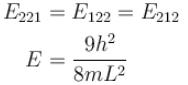3 fold degenerate!

The correct answers are: Ground state energy is non zero, The second excited state is 3 fold degenerate

*Multiple options can be correct
QUESTION: 4

Consider an atomic electron in the l = 3 state. If L is the magnitude of the angular momentum, Lz is its z component, θ is the angle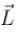makes with the z axis then :

Solution:

l = 3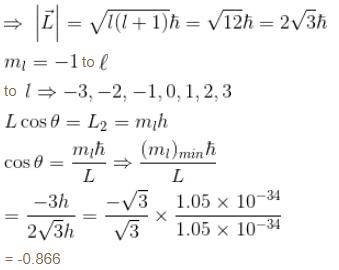The correct answer is: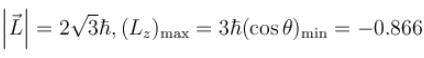*Multiple options can be correct
QUESTION: 5

For a quantum harmonic oscillator in 3 dimensions

Solution:(0, 0, 3)  →   3 state
(1, 1, 1)  →   1 state
(0, 1, 2)  →   6 state

∴   3 + 1 + 6 = 10 fold degenerate.

The correct answers are: The ground state is non degenerate, The third excited state is 10 fold degenerate

*Multiple options can be correct
QUESTION: 6

Consider the case of a 3 dimensional harmonic oscillator. Choose the correct statement.

Solution: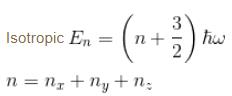can be degenerate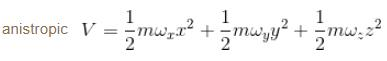No symmetry in potential. Energy levels cannot be degenerate. Both have zero point energy.

The correct answers are: In case of an isotropic harmonic oscillator, the energy levels can be degenerate, In case of an anisotropic oscillator, there is no degeneracy since the potential is not symmetric, Both isotropic and anisotropic oscillators have zero point energy

*Multiple options can be correct
QUESTION: 7

Select the correct statements about the uncertainty principle in 3 dimensions

Solution:

Uncertainty in x = Δx
Uncertainty in px = Δpx

Uncertainty principle in x-direction,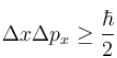Uncertainty principle in y-direction,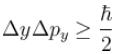Uncertainty principle in z-direction,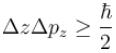No relation between Δx and Δpy and so on.

The correct answers are: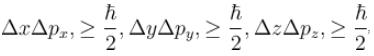No restriction on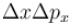and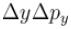and so on

*Multiple options can be correct
QUESTION: 8

Consider the case of particle in a 3d box and a 3d harmonic oscillator.

Solution: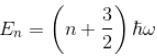(For Harmonic Oscillator)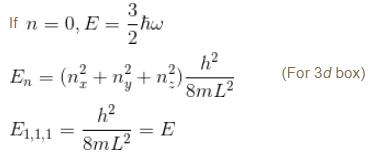The correct answers are: The energy of the ground state of a 3d  harmonic oscillator is zero., The ground state energy of 3d  simple harmonic oscillator is non zero, The ground state energy of a particle in a 3d  box is non zero

*Multiple options can be correct
QUESTION: 9

For a particle in 3 dimensional box

Solution:

The correct answers are: The number of quantum numbers is equal to dimensions of potential distribution, The symmetry of box leads to degeneracy, The spin quantum number does not arise from Schrodinger wave equations, If we consider spin also for fermions then the degeneracy of energy levels become twice

*Multiple options can be correct
QUESTION: 10

For a rectangular 3 dimensional box

Solution:

For a 3d  box with different edge length (ax, ay, az)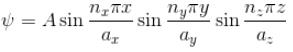ψ will be different even if different combination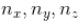gives the same value of En. But the parity is the same

The correct answers are: Degenerate energy levels have different wave functions, Degenerate energy levels have same parity